Area of Parallelogram

# Area of Parallelogram Notes | Study Mathematics (Maths) Class 7 - Class 7

## Document Description: Area of Parallelogram for Class 7 2022 is part of Mathematics (Maths) Class 7 preparation. The notes and questions for Area of Parallelogram have been prepared according to the Class 7 exam syllabus. Information about Area of Parallelogram covers topics like Area of Parallelogram and Area of Parallelogram Example, for Class 7 2022 Exam. Find important definitions, questions, notes, meanings, examples, exercises and tests below for Area of Parallelogram.

Introduction of Area of Parallelogram in English is available as part of our Mathematics (Maths) Class 7 for Class 7 & Area of Parallelogram in Hindi for Mathematics (Maths) Class 7 course. Download more important topics related with notes, lectures and mock test series for Class 7 Exam by signing up for free. Class 7: Area of Parallelogram Notes | Study Mathematics (Maths) Class 7 - Class 7
 1 Crore+ students have signed up on EduRev. Have you?

Area of Parallelogram

The area of a parallelogram is defined as the region or space covered by a parallelogram in a two-dimensional plane. A parallelogram is a special kind of quadrilateral. If a quadrilateral has two pairs of parallel opposite sides, then it is called a parallelogram. Rectangle, square, and rhombus are all examples of a parallelogram. Geometry is all about shapes, 2D or 3D. All of these shapes have a different set of properties with different formulas for area. The prime focus here will be entirely on the following:

• Definition of the area of a parallelogram
• Formula of the area of a parallelogram
• Calculation of a parallelogram's area in vector form

What Is the Area of Parallelogram?

The area of a parallelogram refers to the total number of unit squares that can fit into it and it is measured in square units (like cm2, m2, in2, etc). It is the region enclosed or encompassed by a parallelogram in two-dimensional space. Let us recall the definition of a parallelogram. A parallelogram is a four-sided, 2-dimensional figure with:

• two equal, opposite sides,
• two intersecting and non-equal diagonals, and
• opposite angles that are equal

We come across many geometric shapes other than rectangles and squares in our daily lives. Since few properties of a rectangle and the parallelogram are somewhat similar, the area of the rectangle is similar to the area of a parallelogram.

Area of a Parallelogram Formula
The area of a parallelogram can be calculated by multiplying its base with the altitude. The base and altitude of a parallelogram are perpendicular to each other as shown in the following figure. The formula to calculate the area of a parallelogram can thus be given as,
Area of parallelogram = b × h square units

where,

• b is the length of the base
• h is the height or altitude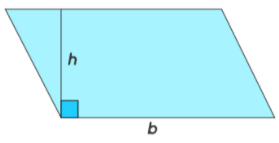Area of Parallelogram, A•bh

Let us analyze the above formula using an example. A assume PQRS is a parallelogram. Using a grid paper, let us find its area by counting the squares.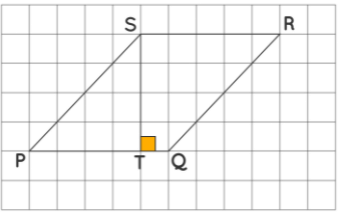From the above figure:
Total number of complete squares = 16
Total number of half squares = 8
Area = 16 + (1/2) × 8 = 16 + 4 = 20 unit2
Also, we observe in the figure that ST ⊥ PQ. By counting the squares, we get:
Side, PQ = 5 units
Corresponding height, ST=4 units
Side x height = 5 × 4 = 20 unit2
Thus, the area of the given parallelogram is base times the altitude.
Let's do an activity to understand the area of a parallelogram.

• Step I: Draw a parallelogram (PQRS) with altitude (SE) on a cardboard and cut it.
• Step II: Cut the triangular portion (PSE).
• Step III: Paste the remaining portion (EQRS) on a white chart.
• Step IV: Paste the triangular portion (PSE) on the white chart joining sides RQ and SP.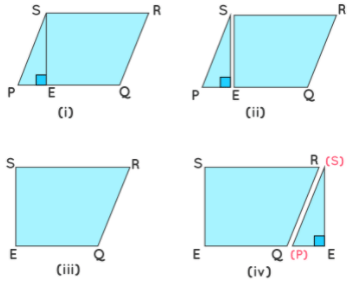After doing this activity, we observed that the area of a rectangle is equal to the area of a parallelogram. Also, base and height of the parallelogram is equal to the length and breadth of the rectangle respectively.
Area of Parallelogram = Base × Height

How To Calculate Area of a Parallelogram?

The parallelogram area can be calculated with the help of its base and height. Also, the area of a parallelogram can also be evaluated if its two diagonals are known along with any of their intersecting angles are known, or if the length of the parallel sides along with any of the angles between the sides is known.

Parallelogram Area Using Height
Suppose 'a' and 'b' are the set of parallel sides of a parallelogram and 'h' is the height (which is the perpendicular distance between 'a' and 'b'), then the area of a parallelogram is given by:
Area = Base × Height
A = b × h [square units]
Example: If the base of a parallelogram is equal to 5 cm and the height is 4 cm, then find its area.
Solution: Given, length of base = 5 cm and height = 4 cm
As per the formula, Area = 5 × 4 = 20 cm2

Parallelogram Area Using Lengths of Sides
The area of a parallelogram can also be calculated without the height if the length of adjacent sides and angle between them are known to us. We can simply use the area of the triangle formula from the trigonometry concept for this case.
Area = ab sin (θ)
where,

• a and b = length of parallel sides, and,
• θ = angle between the sides of the parallelogram.

Example: The angle between any two sides of a parallelogram is 90 degrees. If the length of the two parallel sides is 4 units and 6 units respectively, then find the area.
Solution:
Let a = 4 units and b = 6 units

θ = 90 degrees
Using area of parallelogram formula,
Area = ab sin (θ)
⇒ A = 4 × 6 sin (90º)
⇒ A = 24 sin 90º
⇒ A = 24 × 1 = 24 sq.units.
Note: If the angle between the sides of a parallelogram is 90 degrees, then the parallelogram becomes a rectangle.

Parallelogram Area Using Diagonals
The area of any given parallelogram can also be calculated using the length of its diagonals. There are two diagonals for a parallelogram, intersecting each other at certain angles. Suppose, this angle is given by x, then the area of the parallelogram is given by:
Area = ½ × d× d2 sin (x)

where,

• d1 and d= Length of diagonals of the parallelogram, and
• x = Angle between the diagonals.

Area of Parallelogram in Vector Form

The area of the parallelogram can be calculated using different formulas when either the sides or the diagonals are given in the vector form. Consider a parallelogram ABCD as shown in the figure below,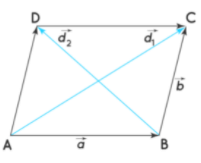Area of parallelogram in vector formArea of parallelogram in vector form using the adjacent sides is,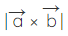where,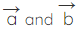are vectors representing two adjacent sides.
Here,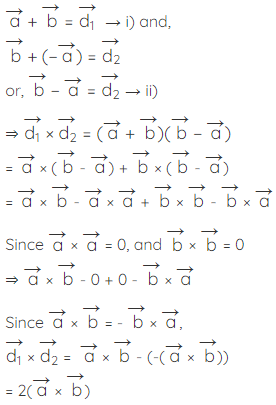Therefore, area of parallelogram when diagonals are given in vector form =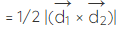where,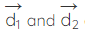are diagonals.

Solved Examples

Example 1: The adjacent sides of a parallelogram are 10 in and 6 in. The altitude corresponding to side 10 in is 5 in. Using the area of parallelogram formula, find the area and the length of the altitude corresponding to its adjacent side.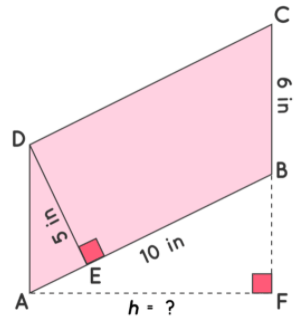Solution: Let ABCD be a parallelogram where DE⊥AB, AF⊥BC
Using the area of parallelogram formula,
Area of Parallelogram ABCD = (10) × (5) = 50 in2
Length of BC = 6 in
Length of Altitude AF = (50 ÷ 6) = 8.3 in

Example 2: Calculate the area of a solar sheet which is in the shape of a parallelogram, given that, the base measures 20 in, and the altitude measures 8 in.
Solution:
Using the area of parallelogram formula,
Area of the solar cell sheet = B × H = (20) × (8) = 160 in2

Example 3: The area of a playground which is in the shape of a parallelogram is 2500 in2, with one side measuring 250 in. Find the corresponding altitude using area of parallelogram formula.
Solution: Area of the playground = 2500 in2
Side of a playground = 250 in
Corresponding altitude = 2500/250 = 10 in

The document Area of Parallelogram Notes | Study Mathematics (Maths) Class 7 - Class 7 is a part of the Class 7 Course Mathematics (Maths) Class 7.
All you need of Class 7 at this link: Class 7

## Mathematics (Maths) Class 7

168 videos|252 docs|45 tests
 Use Code STAYHOME200 and get INR 200 additional OFF

## Mathematics (Maths) Class 7

168 videos|252 docs|45 tests

Track your progress, build streaks, highlight & save important lessons and more!

,

,

,

,

,

,

,

,

,

,

,

,

,

,

,

,

,

,

,

,

,

;